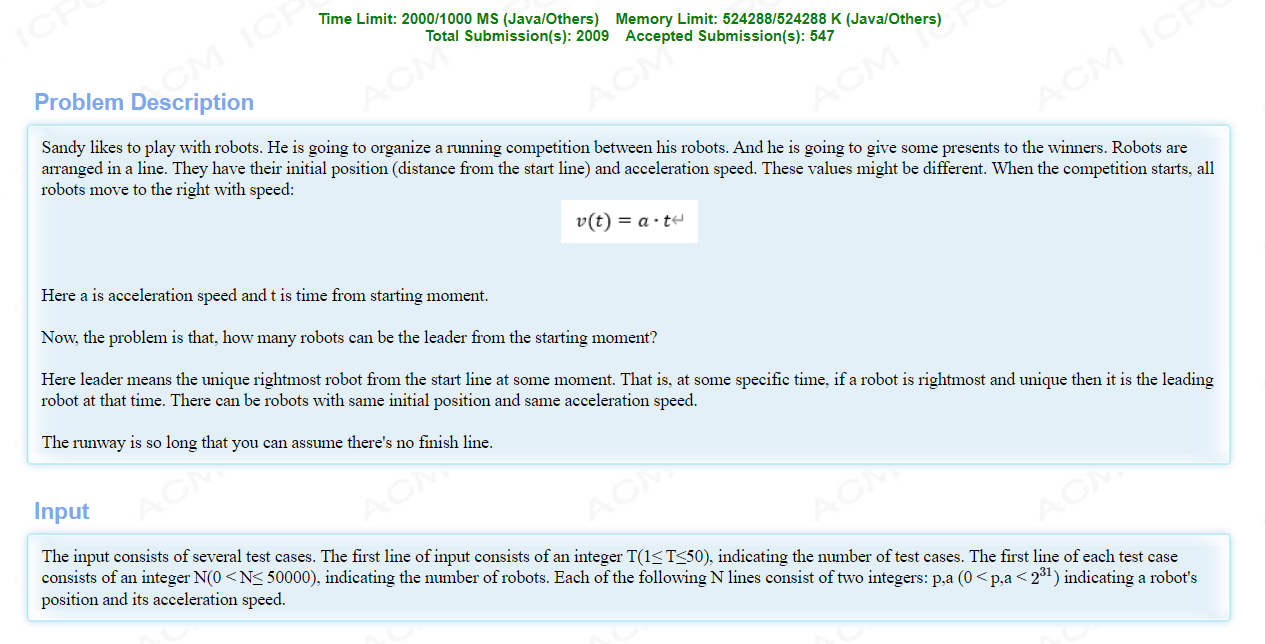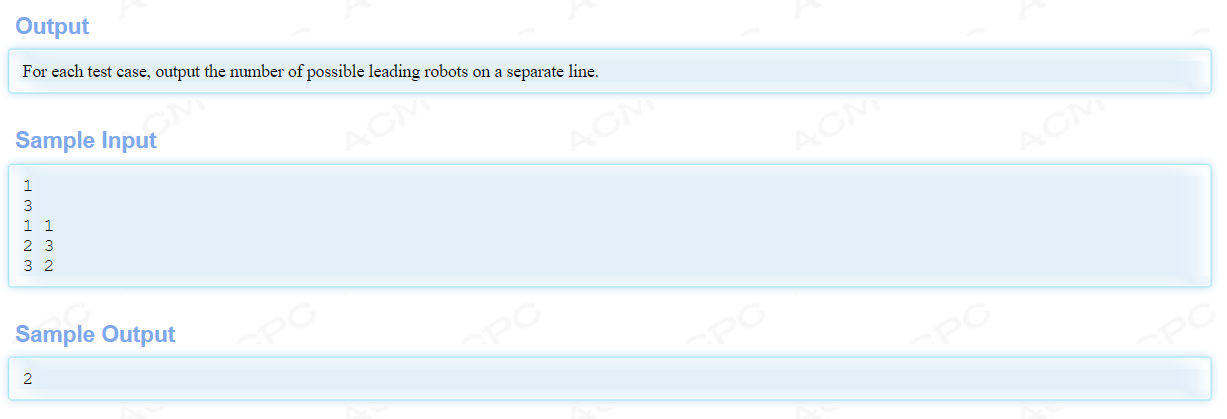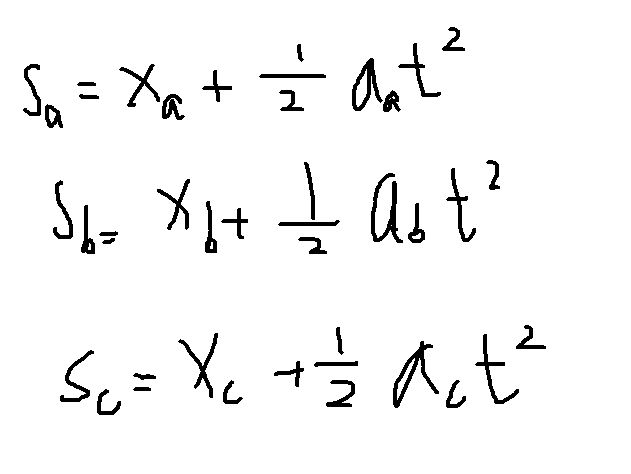1.如果排序之后在后面的，那么如果他的位置大于前面的，那么前面的肯定就没有机会做第一。
2.如果有a,b,c。那么c追上b的条件是b追上a的时间必须小于c追上b的时间。如果大于或者等于，那么b还没有追上a的时候已经被c超过了。所以这时b就没有机会第一。AC代码：

``````#include<bits/stdc++.h>
using namespace std;
typedef long long ll;
const ll maxn=5e4+10;
struct Node{

ll x,a;
}ro[maxn];

bool Judge(Node A,Node B,Node C){

return (B.x-C.x)*(B.a-A.a)-(C.a-B.a)*(A.x-B.x)<=0;
}
bool cmp(Node A,Node B){

if(A.a!=B.a)return A.a<B.a;
else return A.x<B.x;
}
map<pair<ll,ll>,ll> mp;
ll n,top,Stack[maxn];
int main(){

ll T;
scanf("%lld",&T);
while(T--){

mp.clear();//用来确保不重复的
scanf("%lld",&n);
for(ll i=0;i<n;i++){

scanf("%lld %lld",&ro[i].x,&ro[i].a);
pair<ll,ll> p;
p.first=ro[i].a;
p.second=ro[i].x;
mp[p]++;
}
sort(ro,ro+n,cmp);
ll top=0;
for(ll i=0;i<n;i++){

while((top>0&&ro[i].x>=ro[Stack[top]].x)||(top>1&&Judge(ro[Stack[top-1]],ro[Stack[top]],ro[i]))){

//如果top>=0并且ro[i]的位置在顶部元素的后面，那么前面的就不可能得到第一，
top--;
}
Stack[++top]=i;
}
ll ans=top;
for(ll i=1;i<=top;i++){

pair<ll,ll> p;
p.first=ro[Stack[i]].a;
p.second=ro[Stack[i]].x;
if(mp[p]>1)ans--;
}
printf("%lld\n",ans);
}
return 0;
}
``````